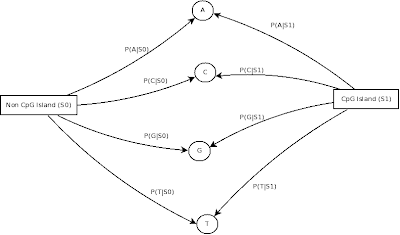## Jun 2, 2011

### CpG Islands (2) - Building a Hidden Markov Model

By the definition of the CpG islands in the previous post and the Hidden Markov Models (HMMs) short introduction, we now can model a HMM for finding CpG islands. We can create a model very similar to the "Fair Bet Casino" problem.

When we are in a nucleotide of given DNA sequence there are two possibilities, that nucleotide belongs to CpG island (lets denote state S1) or do not (S0). If you analyse a sibling nucleotide it can stay in the same state or to change with complementary probabilities. We can view it as this Markov chain:CpG states Markov chain

As the emissions of the states S0 and S1, we may have the associated probabilities of the emissions of ACGT symbols. However we can't know for now how much are these probabilities. However if we have a dataset of sequences with the attached information of where the CpG islands occurs, we can estimate those parameters. I used these DNA sequences tagged by biologists (used biopython library to parse the .fasta files) as the training and test sets to build the model and evaluate the technique. This dataset consists of nucleotides in upper and lower cases. When we have a given nucleotide in upper case, it denotes that it is a CpG site and the lower case nucleotides means they are not. I used a statistical technique called maximum likelihood to build the HMM.

Using the maximum likelihood to calculate the HMM emissions probabilities, I count each char frequency in the dataset and divide by the total amount of nucleotides in the same state:Emissions probability diagram
```P(A|S0) = a / (a+c+g+t) P(C|S0) = c / (a+c+g+t) P(G|S0) = g / (a+c+g+t) P(T|S0) = t / (a+c+g+t) P(A|S1) = A / (A+C+G+T) P(C|S1) = C / (A+C+G+T) P(G|S1) = G / (A+C+G+T) P(T|S1) = T / (A+C+G+T) ```
And to calculate the transition probabilities of each state, count the number of transitions between a CpG to a non CpG site (out_of) and the reversal transitions (into). Then divide each of them by the state frequency which they're coming from. Note that the permanence probability of each state is the complement of the probability of transiting between states since there is no other state to go:
``` P(S0|S1) = out_of / (A+C+G+T) P(S1|S0) = into / (a+c+g+t) P(S0|S0) = 1 - P(S1|S0) P(S1|S1) = 1 - P(S0|S1) ```
Our model is almost done, it just lacks of the initial transitions which can be supposed to be the frequency of each state over all nucleotides:
``` P(S0) = a+c+g+t/(a+c+g+t+A+C+G+T) P(S1) = A+C+G+T/(a+c+g+t+A+C+G+T) ```
I used the ghmm to create my HMM with the given dataset and it generated this model:
``` DiscreteEmissionHMM(N=2, M=4)   state 0 (initial=0.97)     Emissions: 0.30, 0.21, 0.20, 0.29     Transitions: ->0 (0.00), ->1 (1.00)   state 1 (initial=0.03)     Emissions: 0.22, 0.29, 0.29, 0.20     Transitions: ->0 (1.00), ->1 (0.00)```

It's important to notice that when we print the HMM it says that has the probability of 1 to stay in the same state. This occurs due to float formatted print. The reality is that in that value is very close to 1 but still has a chance to hop between states. It's also interesting to see the probability of emission of C and G when we contrast the states. In S1 the probability of getting a C or a G is significantly higher than in S0.

Another manner of creating the HMM, is by initializing a model with the same structure we modelled the problem and then apply some unsupervised learning algorithms, such as the Baum Welch algorithm. The Baum Welch uses randomized initial weights in the HMM and once you feed untagged sequences, it tries to infer a model. However, usually is far better initialize your HMM with biased data about the problem (for example using the model we created) and let the Baum Welch calibrate the probabilities. With ghmm do the following:
``` sigma = ghmm.IntegerRange(0,4) # our emission alphabet emission_seq = ghmm.EmissionSequence(sigma, [0, 4, 5, ... ]) # create a emission sequence hmm.baumWelch(sigma, emission_seq) # use a template hmm to calibrate the probablities```

In the next post I will show how is the evaluation process of the HMM in the CpG island.

#### 3 comments:

1.I read one of your another blog on this that was part 3 and requested for more theory.But here I wanted to say that this much is really enough from examination point of view as well as knowledge.Thanks
electronic signatures

2.I don't suppose the dataset you used for training purposes is still available somewhere? I can't seem to find it at the provided link.

3.This comment has been removed by a blog administrator.